RS Aggarwal Class 10 Solutions Chapter 13 Constructions

RS Aggarwal Class 10 Chapter 13 Constructions Solutions Free PDF

Constructions is an important chapter for class 10 students, which teaches students about the construction of different geometrical shapes with the help of a compass and a protractor. With the help of this chapter, students can learn how to construct different types of angles such as 30,45,60,90 and 120-degree angles. It is a critical concept for students studying mathematics and students can learn how to construct different geometrical figures such as angle bisector, line segment bisector, perpendicular lines, equilateral triangle, pentagon, and hexagon.

Check out the RS Aggarwal Class 10 Solutions Chapter 13 Constructions available below:

Q.1: Draw a line segment AB of length 7cm. Using ruler and compasses find a point P on AB such that $\frac{AP}{AB} = \frac{3}{5}$</amp-mathml/>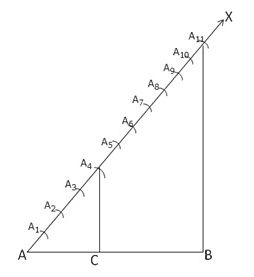Steps of Construction:

Step 1: Draw a line segment AB = 6.5 cm

Step 2: Draw a ray AX making $\angle$ BAX

Step 3: Along AX mark (4 + 7) = 11 points

A1, A2, A3, A4, A5, A6, A7, A8, A9, A10, A11 such that: AA1 = A1A2

Step 4: Join A11 and B.

Step 5: Through A4 draw a line parallel to A11 B meeting AB at C. Therefore, C is the point on AB, which divides AB in the ratio 4 : 7

On measuring,

AC = 2.4 cm

CB = 4.1 cm

Q.2: Draw a line segment of length 7.6cm and divide it in the ratio 5:8. Measure the two parts.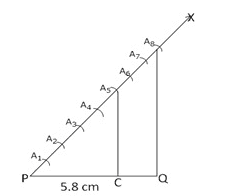Steps of construction:

Step 1: Draw a line segment PQ = 5.8 cm

Step 2: Draw a ray PX making an acute angle QPX.

Step 3: Along PX mark (5 + 3) = 8 points.

A1, A2, A3, A4, A5, A6, A7 and A8 such that:

PA1 = A1A2 = A2A3 = A3A4 =A4A5 = A5A6 = A6A7= A7A8

Step 4: Join A8Q.

Step 5: From A5 draw A5C $\left | \right |$ A8Q meeting PQ at  C.

C is the point on PQ, which divides PQ in the ratio 5 : 3

On measurement,

PC = 3.6 cm, CQ = 2.2 cm

Q.3: Construct a $\triangle$PQR, in which PQ = 6cm, QR = 7cm and PR = 8cm. Then, construct another triangle whose sides are $\frac{4}{5}$ times the corresponding sides $\triangle$PQR.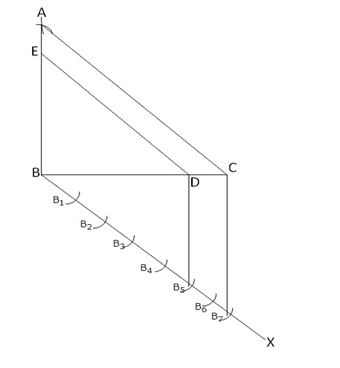Steps of construction:

Step 1: Draw a line segment BC = 6 cm

Step 2: With B as the Center and radius equal to 5 cm draw an arc.

Step 3: With C as the center and radius equal to 7 cm draw another arc cutting the previous arc at A.

Step 4: Join AB and AC. Thus, $\Delta$ ABC is obtained.

Step 5: Below BC draw another line BX.

Step 6: Mark 7 points B1  B2 B3 B4 B5 B6 B7 such that:

BB1 = B1B2 = B2B3 = B3B4 = B4B5 = B5B6 = B6B7

Step 7: Join B7C

Step 8: From B5, draw B5D $\left | \right |$ B7C

Step 9: Draw a line DE through D parallel to CA

Hence $\Delta$ BDE is the required triangle.

Q.4: Construct a triangle with sides 5cm, 6cm and 7cm and then another triangle whose sides are $\frac{7}{5}$ of the corresponding sides of the first triangle.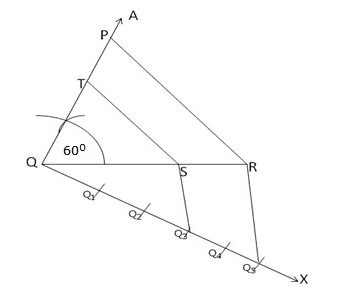Steps of Construction:

Step 1: Draw a line segment QR = 6 cm

Step 2: At Q, draw an angle RQA of 60o

Step 3: From QA cut off a segment QP = 5 cm.

Join PR. $\Delta$ PQR is the given triangle.

Step 4: Below QR draw another line QX.

Step 5: Along QX cut – off equal distances Q1 Q2 Q3 Q4 Q5

QQ1 = Q1Q2 = Q2Q3 = Q3Q4 = Q4Q5

Step 6: Join Q5R

Step 7: Through Q3 draw Q3S $\left | \right |$ Q5R

Step 8: Through S, draw ST $\left | \right |$ PR

$\Delta$ TQS is the required triangle.

Q.5: Construct a $\Delta$ABC with BC = 7cm, ∠B = 60° and AB = 6cm. Construct another triangle whose sides are $\frac{3}{4}$ times the corresponding sides of $\Delta$ABC.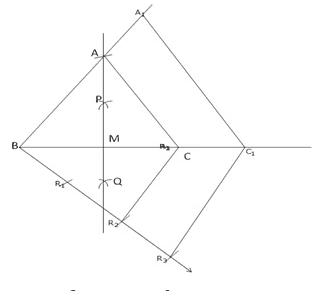Steps for Construction:

Step 1: Draw a line segment BC = 6cm

Step 2: Draw a right bisector PQ of BC meeting it at M.

Step 3: From QP cut – off a distance MA = 4 cm.

Step 4: Join AB, AC.

$\Delta$ ABC is the given triangle.

Step 5: Below BC, draw a line BX

Step 6: Along BX, cut – off 3 equal distances such that

BR1 = R1R2 = R2R3

Step 7: Join R2C

Step 8: Through R3 draw a line R3C1 $\left | \right |$ R2C.

Step 9: Through C1 draw a line C1A1 $\left | \right |$ CA.

$\Delta$ A1BC1 is the required triangle.

Q.6: Construct a $\Delta$ABC in which AB = 6cm, ∠A = 30° and ∠B = 60°. Construct another $\Delta$AB’C’ similar to $\Delta$ABC with base AB’ = 8cm.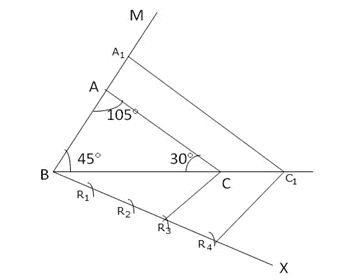Steps of construction:

Step 1: Draw a line segment BC = 5.4 cm

Step 2: At B, draw $\angle$ CBM = 45o

Step 3: Now $\angle$ A = 105o , $\angle$ B = 45 o, $\angle$ C = 180o – (105o + 45o) = 30o

At C draw $\angle$ BCA = 30o

$\Delta$ ABC is the given triangle.

Step 4: Draw a line BX below BC.

Step 5: Cut – off equal distances such that BR1 = R1R2= R2R3 = R3R4

Step 6: Join R3C

Step 7: Through R4, draw a line R4C1 $\left | \right |$ R3C

Step 8: Through C1, draw a line C1A1 parallel to CA

$\Delta$ A1BC1 is the required triangle.

Q.7: Construct a $\Delta$ABC in which BC = 8cm, ∠B = 45° and ∠C = 60°. Construct another triangle similar to $\Delta$ABC such that its sides are $\frac{3}{5}$ of the corresponding sides of $\Delta$ABC.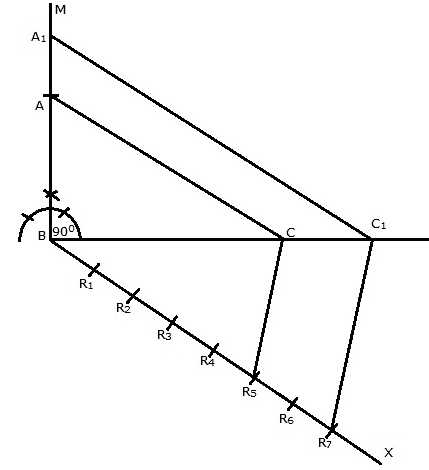Steps of Construction:

Step 1: Draw a line segment BC = 4 cm.

Step 2: Draw a right-angled CBM at B.

Step 3: Cut- off BA = 3 cm from BM.

Step 4: Join AC.

$\Delta$ ABC is the given triangle.

Step 5: Below BC draw a line BX.

Step 6: Along BX, cut- off equal distances such that

BR1 = R1R2 = R2R3 = R3R4 = R4R5 = R5R6 = R6R7

Step 7: Join R5C

Step 8: Through R7 draw a line parallel to R5C cutting BC produced at C1.

Step 9: Through C1 draw a line parallel to CA cutting BA at A1

$\Delta$ A1BC1 is the required triangle.

Q.8: To construct a triangle similar to $\Delta$ABC in which BC = 4.5cm, ∠B = 45° and ∠C= 60°, using a  scale factor of $\frac{3}{7}$, BC will be divided into ratio

(a) 3:4   (b) 4:7  (c) 3:10  (d) 3:7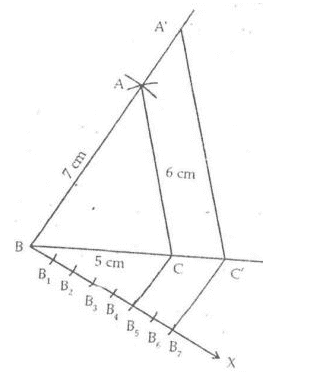Steps of Construction:

Step 1: Draw a line segment BC = 5cm

Step 2: With B as center and radius 7cm an arc is drawn.

Step 3: With C as center and radius 6 cm another arc is drawn intersecting the previous arc at A.

Step 4: Join AB and AC.

Step 5: $\Delta$ ABC is the given triangle.

Step 6: Draw a line BX below BC.

Step 7: Cut – off equal distances from DX such that

BB1 = B1B2 = B2B3 = B3B4 = B4B5 = B5B6= B6B7

Step 8: Join B5C

Step 9: Draw a line through B7 parallel to B5C cutting BC produced at C’.

Step 10: Through C’ draw a line parallel to CA, cutting BA produced at A’.

Step 11: $\Delta$ A’BC’ is the required triangle.

Q.9: Construct an isosceles triangle whose base is 8cm and altitude 4cm and then another triangle whose sides are 1.5 times the corresponding sides of the isosceles triangle.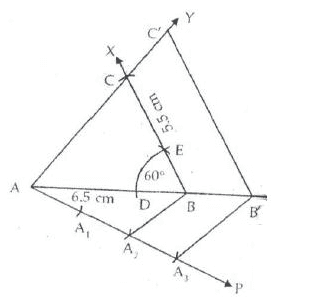Steps of Construction:

Step 1: Draw a line segment AB = 6.5 cm

Step 2: With B as the center and some radius draw an arc cutting AB at D.

Step 3: With center D and some radius draw another arc cutting the previous arc at E. $\angle$ ABE = 60o

Step 4: Join BE and produce it to a point X.

Step 5: With center B and radius 5.5 cm draw an arc intersecting BX at C.

Step 6: Join AC.

$\Delta$ ABC is the required triangle.

Step 7: Draw a line AP below AB.

Step 8: Cut – off 3 equal distances such that

A A1 = A1 A2 = A2 A3

Step 9: Join BA2

Step 10: Draw A3B’ through A3 parallel to A3B

Step 11: Draw a line parallel to BC through B’ intersecting AY at C’.

$\Delta$ AB’C’ is the required triangle.

Q.10: Draw a right triangle in which the sides are of lengths 4cm and 3cm. Then, construct another triangle whose sides are $\frac{5}{3}$ times the corresponding sides of the given triangle.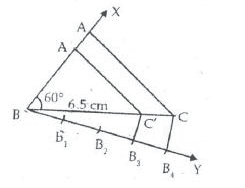Steps of construction:

Step 1: Draw a line segment BC = 6.5 cm

Step 2: Draw an angle of 60o at B so that $\angle$ XBC = 600

Step 3: With center B and radius 4.5 cm, draw an arc intersecting XB at A .

Step 4: Join AC.

$\Delta$ ABC is the required triangle.

Step 5: Draw a line BY below BC.

Step 6: Cut – off 4 equal distances from BY.

Such that BB1 = B1B2 = B2B3 = B3B4

Step 7: Join CB4

Step 8: Draw B3C’ parallel to CB4

Step 9: Draw C’A’ parallel to CA through C’ intersecting BA produced at A’

$\Delta$ A’BC’ is the required similar triangle.

Access CBSE Sample Paper for class 10 Maths here.

Access NCERT Book for class 10 Maths here.

Practise This Question

Male condoms are worn during _______ .